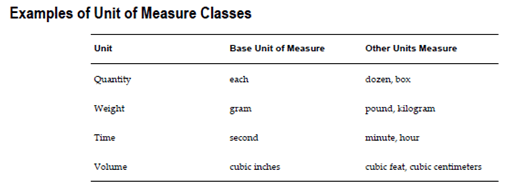# Units of Meas

Oracle Inventory provides you with powerful methods for defining and manipulating units of measure. You can easily convert between the units of measure you define. This enables you to manufacture, order, or receive items in any unit of measure. With units of measure you can:

1. Define unit of measure classes,
2. Define units of measure,
3. Define unit of measure conversions,
4. Define lot-specific unit of measure conversions

Defining Unit of Measure Classes
Unit of measure classes represent groups of units of measure with similar characteristics.

• Creating unit of measure classes is the first step in unit of measure management. Each unit of measure you define must belong to a unit of measure class.
• Each class has a base unit of measure. The base unit of measure is used to perform conversions between units of measure in the class. For this reason, the base unit of measure should be representative of the other units of measure in the class, and generally one of the smaller units. For example, you could use CU (cubic feet) as the base unit of a class called Volume.
• Unit of measure classes are not organization-specific. Default unit of measure classes are not provided.1. Enter a unique name for the unit of measure class.
2. Define the base unit of measure for this class.
3. Define a unique abbreviation for the base unit of measure.0 replies